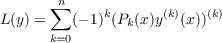#### Vol. 90, No. 1, 1980

 Recent Issues Vol. 325: 1  2 Vol. 324: 1  2 Vol. 323: 1  2 Vol. 322: 1  2 Vol. 321: 1  2 Vol. 320: 1  2 Vol. 319: 1  2 Vol. 318: 1  2Online Archive Volume: Issue:The Journal Subscriptions Editorial Board Officers Contacts Submission Guidelines Submission Form Policies for Authors ISSN: 1945-5844 (e-only) ISSN: 0030-8730 (print) Special Issues Author Index To Appear Other MSP Journals
Comparison and oscillation criteria for selfadjoint vector-matrix differential equations

### Roger T. Lewis and Lynne C. Wright

Vol. 90 (1980), No. 1, 125–134
##### Abstract

Letwhere the coefficients are real, continuous, m × m, symmetric matrices, y(x) is an m-dimensional vector-valued function, and Pn(x) is positive definite for all x I. We consider both the case for which the singularity is at , I = [1,), and the case for which the singularity is at 0, I = (0,1].

The main theorem is a comparison result in which the equation L(y) = 0 is compared with an associated scalar equation. Then, general theorems for the oscillation and nonoscillation of L(y) = 0 are presented which can be used when the comparison theorem does not apply. Some of the proofs indicate how scalar oscillation and nonoscillation criteria can be extended to the vector-matrix case when the associated scalar theorem has been proved using the quadratic functional criteria for oscillation. In general, proofs using the associated Riccati equation and other familiar methods do not extend as easily.

Primary: 34C10
##### Milestones